Courses

# Facts That Matter, Ex 11.1 NCERT Solutions- Constructions Class 10 Notes | EduRev

## Class 10 : Facts That Matter, Ex 11.1 NCERT Solutions- Constructions Class 10 Notes | EduRev

The document Facts That Matter, Ex 11.1 NCERT Solutions- Constructions Class 10 Notes | EduRev is a part of the Class 10 Course Class 10 Mathematics by VP Classes.
All you need of Class 10 at this link: Class 10

Facts that Matter
We know that:
I. To divide a line segment in a given ratio m:n, we divide this segment into (m + n) equal parts. Then we take m parts on one side and n on the other.
II. The idea of dividing a line segment in any ratio is used in construction of a triangle similar to a given triangle, whose sides are in a given ratio with the corresponding sides of the given triangle.
III. The scale-factor means the ratio of the sides of the triangle to be constructed with the corresponding sides of the given triangle.

TANGENTS TO A CIRCLE

Remember:
I. If a point lies inside a circle, then there cannot be a tangent to the circle through this point.
II. If a point lies on the circle, then there is only one tangent to the circle at this point and it is perpendicular to the radius through that point.
III. If the point lies outside the circle, there will be two tangents to the circle from this
point.

NOTE:
(i) For drawing a tangent at a point of a circle, simply draw the radius through this point and draw a line perpendicular to this radius through this point.
(ii) The two tangents to a circle from an external point are equal.

Construction of tangents to a circle from a point outside it.
Steps of construction:
I. Let the centre of the circle be O and P be a point outside the circle.
II. Join O and P.
III. Bisect OP and let M be the midpoint of OP.
IV. Taking M as centre and MP or MO as radius, draw a circle intersecting the given circle at the points A and B.
V. Join PA and PB.
Thus, PA and PB are the required two tangents.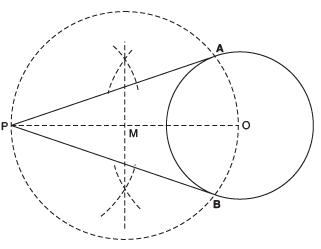NOTE:
In case, the centre of the circle is not known, then to locate its centre, we take any two non-parallel chords and then find the point of intersection of their perpendicular bisectors.

Page No. 219 - 220
EXERCISE 11.1
In each of the following, give the justification of the construction also:
Ques 1: Draw a line segment of length 7.6 cm and divide it in the ratio 5:8.
Measure the two parts.
Sol: Steps of construction:
I. Draw a line segment AB = 7.6 cm.
II. Draw a ray AX making an acute angle with AB.
III. Mark 13 (8 + 5) equal points on AX, and mark them as X1, X2, X3, ........, X13.
IV. Join ‘point X13’ and B.
V. From ‘point X5’, draw X5C ║ X13B, which meets AB at C.
Thus, C divides AB in the ratio 5:8.
On measuring the two parts, we get: AC = 4.7 cm and BC = 2.9 cm.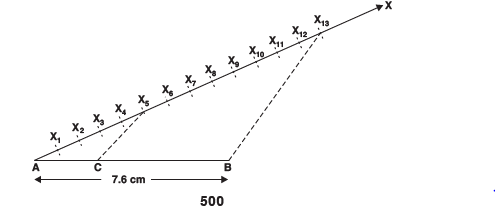Justification:
In Δ ABX13 and Δ ACX5, we have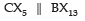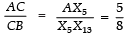⇒ AC : CB = 5 : 8.

Ques 2: Construct a triangle of sides 4 cm, 5 cm and 6 cm and then a triangle similar to it whose sides are 2/3 of the corresponding sides of the first triangle.
Sol: Steps of construction:
I. Draw a ∆ABC such that BC = 6 cm, AC = 5 cm and AB = 4 cm.
II. Draw a ray BX making an acute angle ∠CBX.
III. Mark three points X1, X2, X3 on BX such that BX1 = X1X2 = X2X3.
IV. Join X3C.
V. Draw a line through X2 such that it is parallel to X3C and meets BC at C′.
VI. Draw a line through C′ parallel to CA to intersect BA at A′.
Thus, A′BC′ is the required triangle.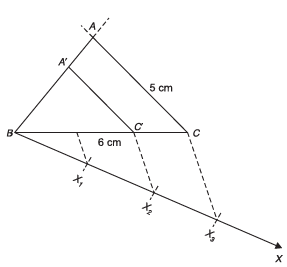Justification:
By construction, we have: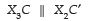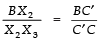But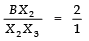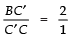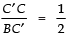Adding, 1 to both sides, we get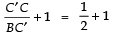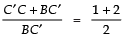⇒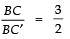Now, in ΔBC′A′ and ΔBCA
we have CA ║ C′A′
∴ Using AA similarity, we have: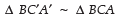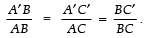[each equal to 2/3]

Ques 3: Construct a triangle with sides 5 cm, 6 cm and 7 cm and then another triangle whose sides are 7/5 of the corresponding sides of the first triangle.
Sol: Steps of construction:
I. Construct a Δ ABC such that AB = 5 cm, BC = 7 cm and AC = 6 cm.
II. Draw a ray BX such that ∠CBX is an acute angle.
III. Mark 7 points of X1, X2, X3, X4, X5, X6 and X7 on BX such that BX1 = X1X2 = X2X3 = X3X4 = X4X5 = X5X6 = X6X7
IV. Join X5 to C.
V. Draw a line through X7 intersecting BC (produced) at C′ such that X5C ║ X7C′.
VI. Draw a line through C′ parallel to CA to intersect BA (produced) at A′. Thus, ΔA′BC′ is the required triangle.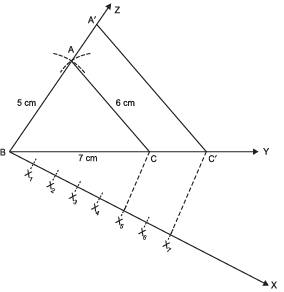Justification:
By construction, we have
C′A′ ║ CA
∴ Using AA similarity, ΔABC ~ ΔA′BC′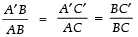Also X7C′ ║ X5C      [By construction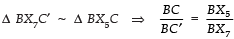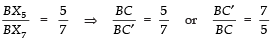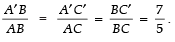Ques 4: Construct an isosceles triangle whose base is 8 cm and altitude 4 cm and then another triangle whose sides are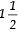times the corresponding sides of the isosceles triangle.
Sol: Steps of construction:
I. Draw BC = 8 cm
II. Draw the perpendicular bisector of BC which intersects BC at D.
III. Mark a point A on the above perpendicular such that DA = 4 cm.
IV. Join AB and AC.
Thus, ΔABC is the required isosceles triangle.
V. Now, draw a ray BX such that ∠CBX is an acute angle.
VI. On BX, mark three points X1, X2 and X3 such that: BX1 = X1X2 = X2X3
VII. Join X2 to C.
VIII. Draw a line through X3 parallel to X2 C and intersecting BC (extended) to C′.
IX. Draw a line through C′ parallel to CA intersecting BA (extended) at A′, thus, ΔA′BC′ is the required triangle.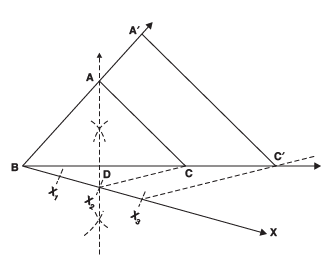Justification:
We have C′A′ ║ CA   [By construction]
∴ Using AA similarity, Δ ABC ~ ΔA′BC′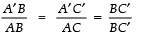Since, X3C′ ║ X2C       [By construction]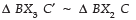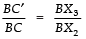[By BPT]
But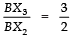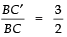Thus,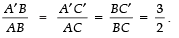Ques 5: Draw a triangle ABC with side BC = 6 cm, AB = 5 cm and ∠ABC = 60°. Then construct a triangle whose sides are 3/4 of the corresponding sides of the triangle ABC.
Sol: Steps of construction:
I. Construct a ΔABC such that BC = 6 cm, AB = 5 cm and ∠ABC = 60°.
II. Draw a ray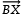such that ∠CBX is an acute angle.
III. Mark four points X1, X2, X3 and X4 on BX such that BX1 = X1X2 = X2X3 = X3X4
IV. Join X4C and draw X3C′ ║ X4C such that C′ is on BC.
V. Also, draw another line through C′ and parallel to CA to intersect BA at A′.
Thus, ΔA′BC′ is the required triangle.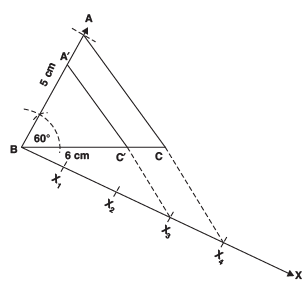Justification:
By construction, we have:
X4C ║ X3C′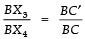[By BPT]
But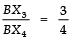[By construction]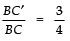...(1)
Now, we also have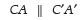[By construction]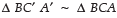[using AA similarity]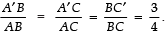[From (1)]

Ques 6: Draw a triangle ABC with side BC = 7 cm, ∠B = 45°, ∠A = 105°. Then, construct a triangle whose sides are 4/3 times the corresponding sides of Δ ABC.
Sol: Steps of construction:
I. Construct a Δ ABC such that BC = 7 cm, ∠B = 45° and ∠A = 105°.
II. Draw a ray BX making an acute angle ∠CBX with BC.
III. On BX, mark four points X1, X2, X3 and X4 such that BX1 = X1X2 = X2X3 = X3X4.
IV. Join X3 to C.
V. Draw X4C′ ║ X3C such that C′ lies on BC (extended).
VI. Draw a line through C′ parallel to CA intersecting the extended line segment BA at A′.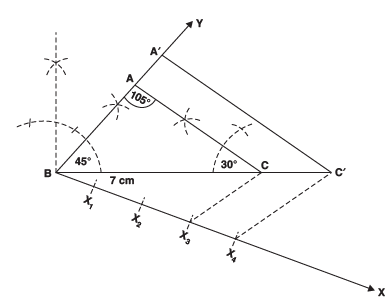Thus, ΔA′BC′ is the required triangle.
Justification:
By construction, we have:
C′A′ ║ CA
∴ ΔABC ~ ΔA′BC′     [AA similarity]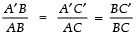...(1)
Also, by construction,
X4C′ ║ X3C
Δ BX4C′ ~ Δ BX3C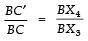But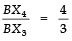⇒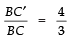..(2)
From (1) and (2), we have: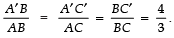Ques 7: Draw a right triangle in which the sides (other than hypotenuse) are of lengths 4 cm and 3 cm. Then construct another triangle whose sides are 5/3 times the corresponding sides of the given triangle.
Sol: Steps of construction:
I. Construct the right triangle ABC such that ∠B = 90°, BC = 4 cm and BA = 3 cm.
II. Draw a ray BX such that an acute angle ∠CBX is formed.
III. Mark 5 points X1, X2, X3, X4 and X5 on BX such that BX1 = X1X2 = X2X3 = X3X4 = X4X5.
IV. Join X3 to C.
V. Draw a line through X5 parallel to X3C, intersecting the extended line segment BC at C′.
VI. Draw another line through C′ parallel to CA intersecting the extended line segment BA at A′.
Thus, ΔA′BC′ is the required triangle.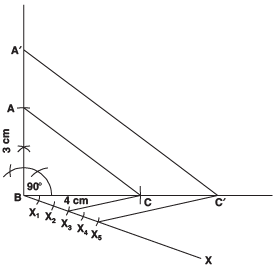Justification:
By construction, we have:
C′A′ ║ CA
∴ ΔABC ~ Δ A′BC′     [By AA similarity ]
⇒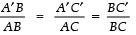...(1)
Also, X5C′ ║ X3C    [By construction]
∴ Δ BX5C′ ~ Δ BX3C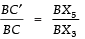But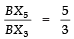...(2)
From (1) and (2) we getOffer running on EduRev: Apply code STAYHOME200 to get INR 200 off on our premium plan EduRev Infinity!

,

,

,

,

,

,

,

,

,

,

,

,

,

,

,

,

,

,

,

,

,

,

,

,

;